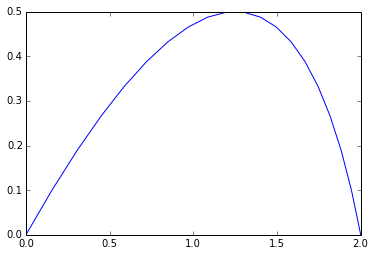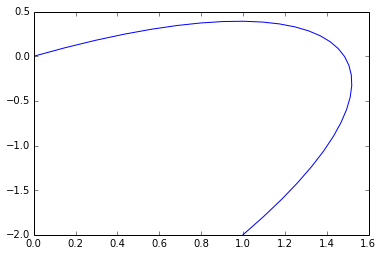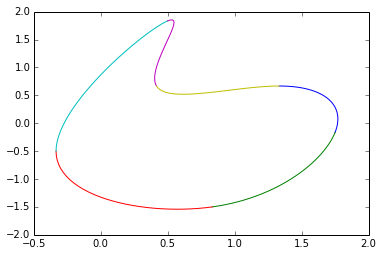Home

So I've been impressed with IPython so far, it can be a little fiddly to install, but given the power I'm not going to complain. I'm now working on graphics and in trying to get up to speed going back and learning, or relearning, some basics. Today was Bézier curves. Note that the below isn't actually educational, you should follow the links provided to really learn about these constructs, I just wanted an excuse to try out LaTeX and plot some interesting graphs.

In :
%pylab inline

Populating the interactive namespace from numpy and matplotlib



The Quadratic Bézier curve is a function of t, where t ranges from 0 to 1.

$\mathbf{B}(t) = (1 - t)^{2}\mathbf{P}_0 + 2(1 - t)t\mathbf{P}_1 + t^{2}\mathbf{P}_2 \mbox{ , } t \in [0,1].$

Where $\mathbf{P}_n$ are the control points. So if we define the function in a way we can use it, and then define some values for the control points:

In :
def B(t):
return (1-t)**2*p0 + 2*t*(1-t)*p1 + t**2*p2

p0 = np.array([0, 0])
p1 = np.array([1.5, 1])
p2 = np.array([2, 0])


We can then plot what that parametrized curve looks like.

In :
xy = np.array([B(t) for t in np.linspace(0, 1, 20)]).T
plot(xy, xy)

Out:
[<matplotlib.lines.Line2D at 0x38de190>]The Cubic Bézier curve has the form of:

$\mathbf{R}(t)=(1-t)^3\mathbf{P}_0+3(1-t)^2t\mathbf{P}_1+3(1-t)t^2\mathbf{P}_2+t^3\mathbf{P}_3 \mbox{ , } t \in [0,1].$

Again, we can define the function and plot it for a given set of points:

In :
def R(t):
return (1-t)**3 * p0 + 3 * t * (1-t)**2 * p1 + 3 * t**2 * (1-t) * p2 + t**3 * p3

In :
p3 = np.array([1, -2])
xy = np.array([R(t) for t in np.linspace(0, 1, 30)]).T
plot(xy, xy)

Out:
[<matplotlib.lines.Line2D at 0x48e5750>]Also interesting are the Uniform cubic B-splines or simply Bezier splines, which can be concatenated smoothly.

In :
def Q(t, p0, p1, p2, p3):
return (((1-t)**3)/(6)) * p0 + ((3*t**3 -6*t**2 + 4)/(6)) * p1 + ((-3*t**3 + 3*t**2 + 3*t + 1)/(6)) * p2 + ((t**3)/(6)) * p3

In :
p4 = np.array([-1, -1])
p5 = np.array([1, 3])


If we now use our 6 points and plot 4 at a time and keep shifting in one new control point then the spline closes itself.

In :
xy = np.array([Q(t, p0, p1, p2, p3) for t in np.linspace(0, 1, 30)]).T
plot(xy, xy)
xy = np.array([Q(t, p1, p2, p3, p4) for t in np.linspace(0, 1, 30)]).T
plot(xy, xy)
xy = np.array([Q(t, p2, p3, p4, p5) for t in np.linspace(0, 1, 30)]).T
plot(xy, xy)
xy = np.array([Q(t, p3, p4, p5, p0) for t in np.linspace(0, 1, 30)]).T
plot(xy, xy)
xy = np.array([Q(t, p4, p5, p0, p1) for t in np.linspace(0, 1, 30)]).T
plot(xy, xy)
xy = np.array([Q(t, p5, p0, p1, p2) for t in np.linspace(0, 1, 30)]).T
plot(xy, xy)

Out:
[<matplotlib.lines.Line2D at 0x50ad2d0>]IPython made all the above possible, you can download the source for this page from here and import it into your own IPython notebook and start working from there. Also, this blog page was generated from that same source with the nbconvert program.

In []: